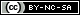# Weibull++ Examples

(Difference between revisions)
 Revision as of 05:51, 15 August 2012 (view source) (→Life Data Analysis)← Older edit Revision as of 06:55, 15 August 2012 (view source)Newer edit → Line 8: Line 8: ==Life Data Analysis== ==Life Data Analysis== - *Simple Probability Plotting Examples: [[Probability Plotting Example|Simple Example]], [[3-Parameter_Weibull_Example|3-P Weibull Example]], [[1P_Exponential_Example|1-P Exponential]], [[Example:_Lognormal_Distribution_Probability_Plot|Lognormal]] + *Simple Probability Plotting Examples: [[Probability Plotting Example|Simple Example]], [[3-Parameter_Weibull_Example|3-P Weibull Example]], [[1P_Exponential_Example|1-P Exponential]], [[Normal Distribution Probability Plotting Example|Normal]], [[Example:_Lognormal_Distribution_Probability_Plot|Lognormal]] *Examples by Distribution: *Examples by Distribution: ::*[[Weibull Distribution Examples|Weibull]] ::*[[Weibull Distribution Examples|Weibull]]

## Life Data Analysis

• Crack Propagation Example (Point Estimation)
View it in HTML or Video

## Recurrent Event Data Analysis

View it in HTML or Video

## Warranty Data Analysis

View it in HTML or Video

## UtilitiesContent on this site is available/licensed under the "Creative Commons Attribution-NonCommercial-ShareAlike 4.0 International License." See Copyright Information for details.